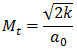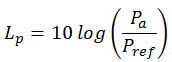SOLIDWORKS 2020 Launch Broadcast, on Tue, Oct 1 at 9:00 AM ET  REGISTER NOW ›

# SOLIDWORKS Flow Simulation 2018 adds Noise PredictionArticle by Siavash Khajehhasani created/updated November 10, 2017

Noise prediction capability is available in SOLIDWORKS Flow Simulation 2018.

The ‘Acoustic Power’ option activates the calculation of a noise level prediction. This acoustic power level uses the “Proudman’s formula” for calculation. This formula is one of the known steady-state approaches in Computational Fluid Dynamics.

Proudman’s approach yield acoustic power due to unit volume of isotropic turbulence in W/mas:Equation (1)

Where u and l are the turbulence viscosity and length scales, respectively. Also, αis the speed of sound and α  in equation (1) is a model constant. Equation (1) can be rewritten as follows in terms of k and e (epsilon):Equation (2)

Wherein,Equation (3)

The rescaled constant of αε is considered as 0.1 in SOLIDWORKS Flow Simulation based on the calibration of Sarkar and Hussaini.

SOLIDWORKS Flow Simulation can also report the acoustic power in dB, which is computed from equation (4):Equation (4)

Wherein Pref  is the reference acoustic power and considered as 1.00e-12 W/m3.

Note: the noise prediction added to Flow Simulation package as of 2017 SP.3 version.

### Posts related to 'SOLIDWORKS Flow Simulation 2018 adds Noise Prediction'### Siavash Khajehhasani

Siavash is a SOLIDWORKS Elite Applications Engineer and is based in our Oakville, Ontario office.

## Want to learn SOLIDWORKS?

Take a training course from our team of Certified SOLIDWORKS Experts